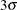#### Example 9.5 Computing Average Run Lengths

See MACEW6 in the SAS/QC Sample LibraryThe EWMAARL DATA step function computes the average run length for an exponentially weighted moving average (EWMA) scheme (refer to Crowder 1987a,b for details). You can use this function to design a scheme by first calculating average run lengths for a range of values for the weight and then choosing the weight that yields a desired average run length.

The following statements compute the average run lengths for shifts between 0.5 and 2 and weights between 0.25 and 1. The data set ARLs is displayed in Output 9.5.1.

data arls;
do shift=.5 to 2 by .5;
do Weight=.25 to 1 by .25;
arl=ewmaarl(shift,Weight,3.0);
output;
end;
end;
run;


Output 9.5.1: Listing of the Data Set ARLs

 Average Run Lengths for Various Shifts and Weights

Weight arl
0.25 48.453
0.50 75.354
0.75 110.950
1.00 155.224

Weight arl
0.25 11.1543
0.50 15.7378
0.75 25.6391
1.00 43.8947

Weight arl
0.25 5.4697
0.50 6.1111
0.75 8.7201
1.00 14.9677

Weight arl
0.25 3.61677
0.50 3.46850
0.75 4.15346
1.00 6.30296

Note that when the weight is 1.0, the EWMAARL function returns the average run length for a Shewhart chart for means. For more details, see EWMAARL Function.

In addition to using the EWMAARL function to design a EWMA scheme with desired average run length properties, you can use it to evaluate an existing scheme. For example, suppose you have an EWMA chart withcontrol limits using a weight parameter of 0.3. The following DATA step computes the average run lengths for various shifts using this scheme:

data ARLinfo;
do shift=0 to 2 by .25;
arl = ewmaarl(shift,0.3,3.0);
output;
end;
run;


The data set ARLinfo is displayed in Output 9.5.2.

Output 9.5.2: Listing of the Data Set ARLinfo

 Average Run Lengths for EWMA Scheme (k=3 and r=0.3)

shift arl
0.00 465.553
0.25 178.741
0.50 53.160
0.75 21.826
1.00 11.699
1.25 7.525
1.50 5.447
1.75 4.258
2.00 3.506